### Metals and Non-metals - Revision Notes

CBSE CLass 10 Science

Revision Notes
CHAPTER – 3
METALS AND NON-METALS

• About 118 elements are known today. There are more than 90 metals,22 non metals and a few metalloids.
• Sodium (Na), potassium (K), magnesium(Mg), aluminium(Al),calcium(Ca), Iron(Fe), Barium(Ba) are some metals.
• Oxygen(O), hydrogen(H), nitrogen(N), sulphur(S), hosphorus(P),fluorine(F), chlorine(Cl), bromine(Br), iodine(l) are some non-metals.
 Metals Non-metals 1. Generally solid except Hg(present in liquid form). 1. Can be solid, liquid organs e.g., C is solid, Br (liq), H2${}_{2}$(gas) 2. Ductile, Malleable (drawn into wires) (beaten into sheets) 2. Non-ductile, non-Malleable 3. Sonorous (produces sound) 3. Non-sonorous 4. Lustrous (have natural shine) 4. Non-lustrous except Iodine. 5. High Melting Point except Ce and Ga 5. Lower M.P. than metals. 6. Generally good conductors of heat and electricity except Pb and Hg. 6. Bad conductors of heat and electricity except Graphite (form of C) 7. High density except Na and K 7. Low densities except Diamond (form of C) 8. Reactive 8. Not very reactive. 9. Ionic bonding is present, 9. Covalent/Hydrogen bonding is present
• Metals form basic oxides e.g., Magnesium oxide(MgO), while non-metals form acidic oxides e.g., $S{O}_{2},C{O}_{2}$ .
• Ag and Cu are best conductors of electricity.
• Metals and Non-metals can be distinguished on the basis of their physical and chemical properties.
• Some elements show the properties of both metals and non-metals and are called metalloids.

Chemical Properties of Metals Reaction with air : Different metals show different reactivities towards oxygen present in air. $Metal+oxygen\stackrel{}{\to }$Metal Oxide

• Some metals like Na and K are kept immersed in kerosene oil as they react vigorously with air and catch fire.
• Some metals like Mg, Al, Zn, Pb react slowly with air and form a protective layer.
• Mg can also burning air with a white dazzling light to form its oxide
• Fe and Cu don’t burn in air but combine with oxygen to form oxide.Iron filings burn when sprinkled over flame.
• Metals like silver, platinum and gold don't burn or react with air.

e.g.. $2Na+\frac{1}{2}{O}_{2}\stackrel{}{\to }N{a}_{2}O$
$2Mg+{O}_{2}\stackrel{}{\to }2MgO$
$2Cu+{O}_{2}\stackrel{}{\to }2CuO$
$4Al+3{O}_{2}\stackrel{}{\to }2A{l}_{2}{O}_{3}$

Usually metal oxides are basic in nature,but some metal oxides show both acidic and basic nature. Amphoteric Oxides :metal oxides which react with both acids as well as bases to form salt and water e.g. $A{l}_{2}{O}_{3,\phantom{\rule{thickmathspace}{0ex}}}ZnO.$
eg.. $A{l}_{2}{O}_{3}+HCl\stackrel{}{\to }AlC{l}_{3}+{H}_{2}O$
$A{l}_{2}{O}_{3}+NaOH\stackrel{}{\to }NaAl{O}_{3}+{H}_{2}O$

REACTION WITH WATER : Metal oxides on reaction with water form alkalis.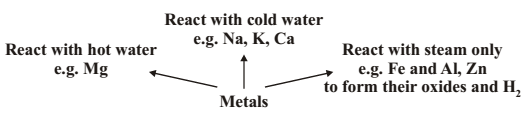$Na+H{O}_{2}\stackrel{}{\to }NaOH+{H}_{2}$

$K+H{O}_{2}\stackrel{}{\to }KOH+{H}_{2}$

$Ca+H{O}_{2}\stackrel{}{\to }Ca\left(OH{\right)}_{2}+{H}_{2}$

$Mg+{H}_{2}O\stackrel{}{\to }Mg\left(OH{\right)}_{2}+{H}_{2}$

In case of Ca and Mg, the metal starts floating due to bubbles of hydrogen gas sticking to its surface.

$Al+{H}_{2}O\stackrel{}{\to }A{l}_{2}{O}_{3}+{H}_{2}$

$Fe+{H}_{2}O\stackrel{}{\to }F{e}_{3}{O}_{4}+{H}_{2}$

Inert metals like Au and Ag do not react with water.

Note: Try Balancing the above Chemical equations yourself

REACTION WITH ACIDS
Metal +dilute acid$\stackrel{}{\to }$ Salt + Hydrogen gas

metals react with dilute hydrochloric acid and dilute sulphuric acid to form chlorides. $Fe+2HCL\to FeC{l}_{2}+{H}_{2}$

$Mg+2HCl\to MgC{l}_{2}+{H}_{2}$

$Zn+2HCl\to ZnC{l}_{2}+{H}_{2}$

$2Al+6HCl\to 2AlC{l}_{3}+3{H}_{2}$

Note : Copper, mercury and silver don’t react with dilute acids.

Hydrogen gas produced is oxidised to water. This happens because $HN{O}_{3}$is a strong oxidising agent when metals react with nitric acid$\left(HN{O}_{3}\right).$ But Mg and Mn, react with very dilute nitric acid to evolve hydrogen gas. 4. Reaction of metals with other metal salts :

All metals are not equally reactive. Reactive metals can displace less reactive metals from their compounds in solution. This forms the basis of reactivity series of metals. Reactivity series is a list of metals arranged in order of their decreasing activities.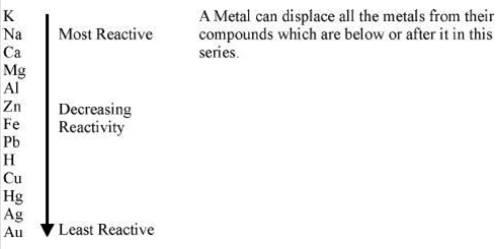$Fe+CuS{O}_{4}\to FeS{O}_{4}+Cu$ $Zn+CuS{O}_{4}\to ZnS{O}_{4}+Cu$ REACTION OF NON-METALS :

 reaction with oxygen non-metals form acidic oxides Eg:C+O2 ->CO2 reaction with water non-metals do not react with water because they cannot release electrons. reaction with dilute acids no reaction reaction with salt solutions a more reactive non-metal will displace less reactive non-metal from its salt solution. reaction with chlorine chloride is formed. Eg;H2(g)+Cl2->2HCl reactions with hydrogen hydrides are formed. H2 + S(l)->H2S

Reaction between Metals and Non-Metals

• Reactivity of elements can be understood as a tendency to attain a completely filled valence shell.
• Atom of metals can lose electrons from valence shells to form cations(+ve ions).
• Atom of non-metals gain electrons in valence shell to form anions (–ve ions).
• Oppositely charged ions attract each other and are held by strong electrostatic forces of attraction forming ionic compounds.

Formation of $MgC{l}_{2}$ $Mg\stackrel{}{\to }M{g}^{2+}+2{e}^{-}$ 2,8, 22,8 (Magnesium ion)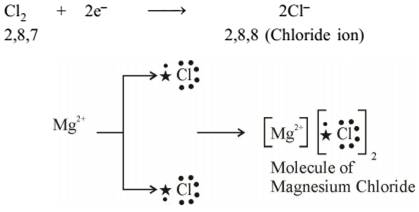Properties of Ionic Compounds

• Are solid and mostly brittle.
• Have high melting and boiling points. More energy is required to break the strong inter-ionic attraction.
• Generally soluble in water and insoluble in kerosene, petrol.
• Conduct electricity in solution and in molten state. In both cases, free ions are formed and conduct electricity.

Occurrence of Metals Minerals : Elements or compounds occurring naturally are minerals. ORES : Mineral from which metal can be profitably extracted is an ore. For example, sulphide ore, oxide ore, carbonate ore.

• Metals at the bottom of activity series like gold, platinum, silver, copper generally occur in free state. But copper and silver also occur as sulphide and oxide ores.
• Metals of medium reactivity (Zn, Fe, Pb etc.) occur mainly as oxides,sulphides or carbonates.
• Metals of high reactivity (K, Na, Ca, Mg and Al) are very reactive and are thus found in combined state.

GANGUE : the commercially valueless materiallike soil,sand, etc. in which ore is found.called gangue. The gangue is removed from the ore. Various Methods to remove gangue: 1.GRAVITY SEPARATION 2.FROTH FLOATATION 3.MAGNETIC SEPARATION METALLURGY : Step-wise process of obtaining metal from its ore.
I. *Enrichment of ore
II. *Obtaining metal from enriched ore.
III. *Refining of impure metal to obtain pure metal.
Enrichment of Ores : It is the process of the removal of impurities such as soil, sand etc. from the ore prior to extraction of the metal. Different separation techniques are used based on physical or chemical properties of ore. Extracting Metals from the Enriched Ore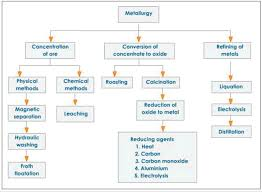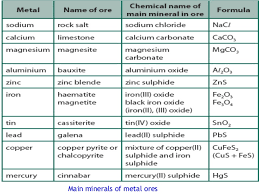Extracting Metals Low in the Activity Series : By heating the ores in air at high temperature. e.g.*Mercury from cinnabar $2HgS+3{O}_{2}\stackrel{Heat}{\to }2HgO+2S{O}_{2}$ $2HgO\stackrel{Heat}{\to }2Hg+{O}_{2}$ e.g. *Copper from copper sulphide $C{u}_{2}S+3{O}_{2}\stackrel{Heat}{\to }2C{u}_{2}{O}_{2}S{O}_{2}$ $2C{u}_{2}S+C{u}_{2}S\stackrel{Heat}{\to }6Cu+S{O}_{2}$ Extracting Metals in the Middle of Activity Series : *Metals are easier to obtain from oxide ores, thus, sulphide and carbonate ores are converted into oxides. *Metal ore heated strongly in excess of air (Roasting) e.g..$2ZnS+3{O}_{2}\stackrel{Heat}{\to }2ZnO+2S{O}_{2}$

Metal ore heated strongly in limited or no supply of air (Calcination) e.g..$ZnS{O}_{3}\stackrel{Heat}{\to }ZnO+C{O}_{2}$

Reduction of Metal Oxide :

USING COKE : Coke as a reducing agent.

$ZnO+C\stackrel{Heat}{\to }Zn+CO$

USING DISPLACEMENT REACTION : highly reactive metal like Na, Ca and Al are used to displace metals of lower reactivity from their compounds. These displacement reactions are highly exothermic. $Mn{O}_{2}+4Al\stackrel{Heat}{\to }3Mn+2A{l}_{2}{O}_{3}+heat$ $F{e}_{2}{O}_{3}+2Al\stackrel{Heat}{\to }2Fe+A{l}_{2}{O}_{3}+heat$

Thermite Reaction : Reduction of a metal oxide to form metal by using Al powder as a reducing agent. This process is used to join broken pieces of heavy iron objects or welding. Extracting Metals at the Top of Activity Series

• These metals have more affinity for oxygen than carbon so they cannot be obtained from their compounds by reducing with carbon.
• So are obtained by electrolytic reduction. e.g.Sodium is obtained by electrolysis of its molten chloride $NaCl\stackrel{}{\to }N{a}^{+}\phantom{\rule{thickmathspace}{0ex}}+\phantom{\rule{thickmathspace}{0ex}}C{l}^{-}$

As electricity is passed through the solution metal gets deposited at cathode and non-metal at anode. At cathode : e.g. $N{a}^{+}+{e}^{-}\stackrel{}{\to }Na$ At anode : $2C{l}^{-}\stackrel{}{\to }C{l}_{2}+2{e}^{-}$  III. Refining of Metals Impurities present in the obtained metal can be removed by electrolytic refining. Copper is obtained using this method. Following are present inside the electrolytic tank. Anode – slab of impure copper Cathode– slab of pure copper Solution – aqueous solution of copper sulphate with some dilute sulphuric acid From anode copper ions are released in the solution and equivalent amount of copper from solution is deposited at cathode. Insoluble impurities containing silver and gold gets deposited at the bottom of anode as anode mud.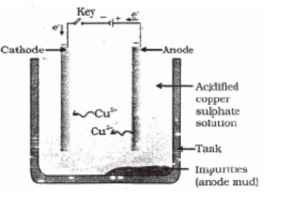Corrosion Metals are attacked by substances in surroundings like moisture and acids. Silver - it reacts with sulphur in air to our form silver sulphide and articles become black. Copper - reacts with moist carbon dioxide in air and gains a green coat of copper carbonate. Iron-acquires a coating of a brown flaky substance called rust. Both air and moisture are necessary for rusting of iron. Rust is hydrated Iron (III) oxide i.e. $F{e}_{2}{O}_{{3}^{.}}x{H}_{2}O$

Prevention of Corrosion Rusting of iron is prevented by painting, oiling, greasing, galvanizing, chrome plating, anodising and making alloys.

In galvanization, iron or steel is coated with a layer of zinc because oxide thus formed is impervious to air and moisture thus protects further layers from corrosion.

Alloys: These are homogeneous mixture of metals with metals or non-metals. Adding small amount of carbon makes iron hard and strong.

Some examples of alloys are following ;
1. Steel : Hard Iron and carbon.Used for construction of roads, railways, other infrastructure, appliances

2. Stainless steel :Hard Rust Free Iron, nickel and chromium. Used in utensils.

3. Brass :Low electrical conductivity Copper and zinc.used for decoration for its bright gold-like appearance and in locks,gears ,plumbing and electrical appliances.

4. Bronze: than pure metal Copper and tin. used to make coins, springs, turbines and blades. 5. Solder : Low MP, used to weld wires Lead and tin. used to create a permanent bond between metal work pieces

6. Amalgam :Used by dentists. Mercury and any other metal

What you have learnt

• Elements can be classified as metals and non-metals.
• Metals are lustrous, malleable, ductile and are good conductors of heat and electricity. They are solids at room temperature, except mercury which is a liquid.
• Metals can form positive ions by losing electrons to non-metals.
• Metals combine with oxygen to form basic oxides. Aluminium oxide and zinc oxide show the properties of both basic as well as acidic oxides. These oxides are know as amphoteric oxides.
• Different metals have different reactivities with water and dilute acids.
• A list of common metals arranged in order of their decreasing reactivity is known as an activity series.
• Metals above hydrogen in the Activity series can displace hydrogen from dilute acids.
• A more reactive metal displaces a less reactive metal from its salt solution.
• Metals occur in nature as free elements or in the form of their compounds.
• The extraction of metals from their ores and then refining them for use is know as metallurgy.
• An alloy is a homogeneous mixture of two or more metals, or a metal and anon-metal.
• The surface of some metals, such as iron, is corroded when they are exposed to moist air for a long period of time. This phenomenon is known as corrosion.
• Non-metals have properties opposite to that of metals. They are neither malleable nor ductile. They are bad conductors of heat and electricity, except for graphite,which conducts electricity.
• Non-metals form negatively charged ions by gaining electrons when reacting with metals.
• Non-metals form oxides which are either acidic or neutral.
• Non-metals do not displace hydrogen from dilute acids. They react with hydrogen to form hydrides.